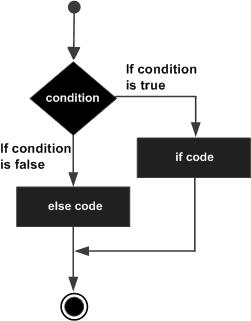# Powershell-If Else声明

## 句法

```if（Boolean_expression）{
//在布尔表达式为true时执行
}其他{
//当布尔表达式为false时执行
}
```

## 流程图## 例

```\$x = 30

if(\$x -le 20){
write-host("This is if statement")
}else {
write-host("This is else statement")
}```

## 输出量

```This is else statement
```

## if … elseif … else语句

if语句后可以是一个可选的else if … else语句，这对于使用单个if … elseif语句测试各种条件非常有用。

• 一个if可以有零个或另一个，并且必须在其他if之后。
• 一个if可以具有零个或多个elseif，并且它们必须位于else之前。
• 如果else if成功，则不会测试其余的elseif。

## 句法

```if(Boolean_expression 1) {
// Executes when the Boolean expression 1 is true
}elseif(Boolean_expression 2) {
// Executes when the Boolean expression 2 is true
}elseif(Boolean_expression 3) {
// Executes when the Boolean expression 3 is true
}else {
// Executes when the none of the above condition is true.
}
```

## 例

```\$x = 30

if(\$x -eq 10){
write-host("Value of X is 10")
} elseif(\$x -eq 20){
write-host("Value of X is 20")
} elseif(\$x -eq 30){
write-host("Value of X is 30")
} else {
write-host("This is else statement")
}```

## 输出量

`Value of X is 30`

• 回顶